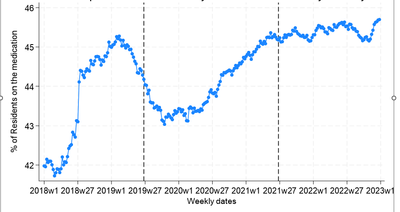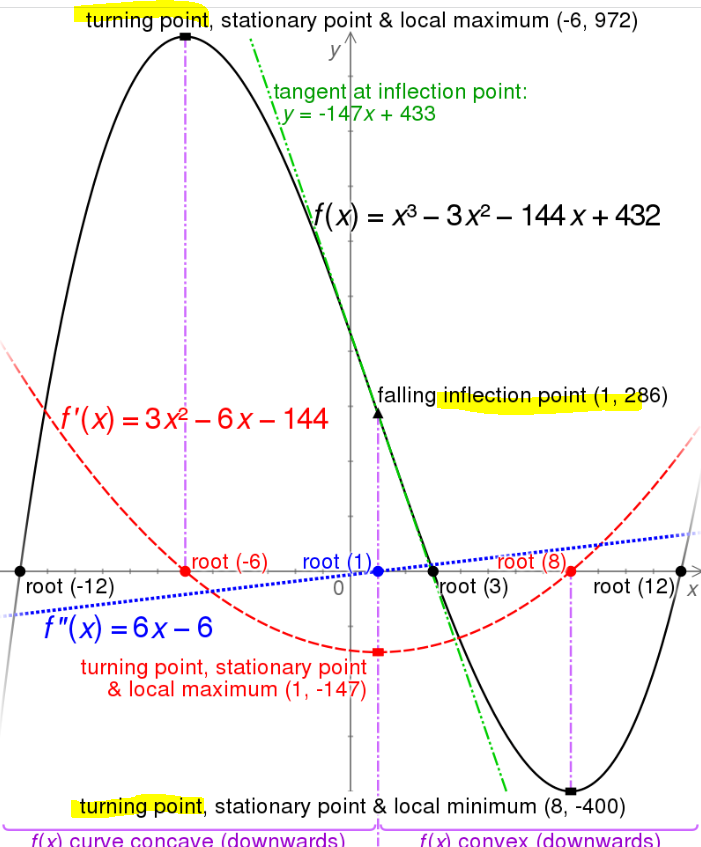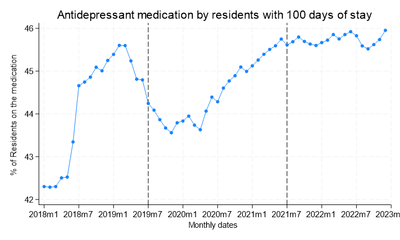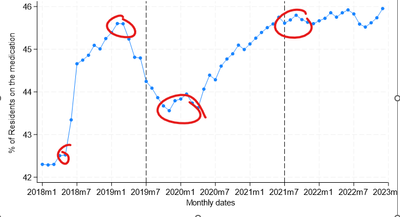## Change of slope in a curve

Hi,
I have a clustered count data where I have counts and denominator within each cluster for a period of 80 months. In order to examine the effect of month on the rate I fitted a random intercept Poisson model. However, the relationship is very non-linear as shown in the plot of raw rates below.Can you please suggest how can I identify the time points at which the slope of the curve is changing (turning points)?

22 REPLIES 22Ksharp
Super User

## Re: Change of slope in a curve

I don't have a suggestion, but I think your question might be slightly different.

Is your question really "how can I fit a model to this sort of data?".   Meaning if you could fit a model to it that you liked, then it would be straight forward to find the points where the slope of the model changes.

As shown in the blog post linked by @Ksharp , there are model-free ways to find local maxima and minima.  I think in that context for this sort of data with some noise in it, you would need to think about how to empirically define the point of a slope change aka local min/max aka vertex (?).  For example, perhaps think about how many "slope change" points you see on the plot, and then think backwards to a reasonable rule that would help identify those points.

Check out the Boston Area SAS Users Group (BASUG) video archives: https://www.basug.org/videos.

## Re: Change of slope in a curve

By "turning point" do you mean "inflection point" ?  https://en.wikipedia.org/wiki/Inflection_point

Inflection point has a standard usage but I am afraid "turning point" means nothing to me.

And if "month" is the desired "effect" on rate , not "month of year", then perhaps you need to look at things differently. And if the topic is "month" why does the graph use week for tick marks???

Several of the Timeseries related procedures deal with "seasonality" such as month in different years. Perhaps that is where you need to look.

## Re: Change of slope in a curve

Perhaps I misunderstood, but I was interpreting "turning point" as being a local minimum or maximum, where the sign of the slope changes.   I haven't heard this term before, but an image on the wikipedia page you linked to uses "turning point" as a label for local minima and maxima.Edit:  I found a wikipedia page with a definition for turning point, consistent with my guess: "turning point is a point at which the derivative changes sign."

https://en.wikipedia.org/wiki/Stationary_point

Check out the Boston Area SAS Users Group (BASUG) video archives: https://www.basug.org/videos.

## Re: Change of slope in a curve

Hi,
The term "turning point" may not be statistically appropriate. It should be "Change in trend". It is not only month but month of the year. I analysed data with both weekly and monthly resolution and by mistake I put the weekly graph. The monthly graph is given below.
During the period between 2018 and 2022 few intervention happened, whose exact timing is unclear, and they are supposed affect the trend of the rate of medication use. I need to identify those time points.## Re: Change of slope in a curve

Can you highlight on the plot the points you would want to identify as "change in trend"?  Just by eyeballing.

Check out the Boston Area SAS Users Group (BASUG) video archives: https://www.basug.org/videos.

## Re: Change of slope in a curve## Re: Change of slope in a curve

One possible solution is to define a piecewise linear regression model.

If you know the time at which the change occurs, you can solve the problem by using a piecewise linear spline and setting the "knot locations" to be the known times. See https://blogs.sas.com/content/iml/2017/04/05/nonsmooth-models-spline-effects.html

If you do not know the change points, then you have to solve for them. These are known as segmented regression models. For an example of a single change point, see https://blogs.sas.com/content/iml/2020/12/14/segmented-regression-sas.html

## Re: Change of slope in a curve

Hi,
I do not know the time points when the changes occur and there are multiple of this. Is it possible to fit a Generalised Additive Model (GAM) to determine the smooth function and then find out the maximas and minimas by taking the derivatives?

Br, Koen

## Re: Change of slope in a curve

Rajesh gave an excellent presentation to BASUG last year on PROC SSM and PRO UCM.  Slides and recording are in our archives: https://www.basug.org/archives

Check out the Boston Area SAS Users Group (BASUG) video archives: https://www.basug.org/videos.

## Re: Change of slope in a curve

> Is it possible to fit a GAM to determine the smooth function and then find out the maximas and minimas by taking the derivatives?

Yes, it is possible, if that is what you want to do. If you evaluate any model (GAM, loess, spline, MARS,...) at points on a fine, evenly spaced grid for the independent variable, then you can use numerical differentiation to estimate the extrema. See Find points where a regression curve has zero slope - The DO Loop (sas.com)

## Re: Change of slope in a curve

Hi,
Thanks for the link. Can we find the confidence interval of the identified points?

## Re: Change of slope in a curve

> Can we find a confidence interval of the identified points?

You could if you use a segmented regression model or some other rigorous statistical model. But if you fit some smoother to the data and find places where the derivative of the smoother is 0, I don't think you can get a CI automatically from the regression. For one thing, different smoothers will give you different result s. (For example, think about a loess smoother with different smoothing parameters!)

I think the fit-a-smoother-and-take-derivative method is a heuristic method that doesn't have much statistical theory behind it. If you want something like a confidence interval, you need a parameter in a statistical model.

Discussion stats
• 22 replies
• 919 views
• 3 likes
• 6 in conversation